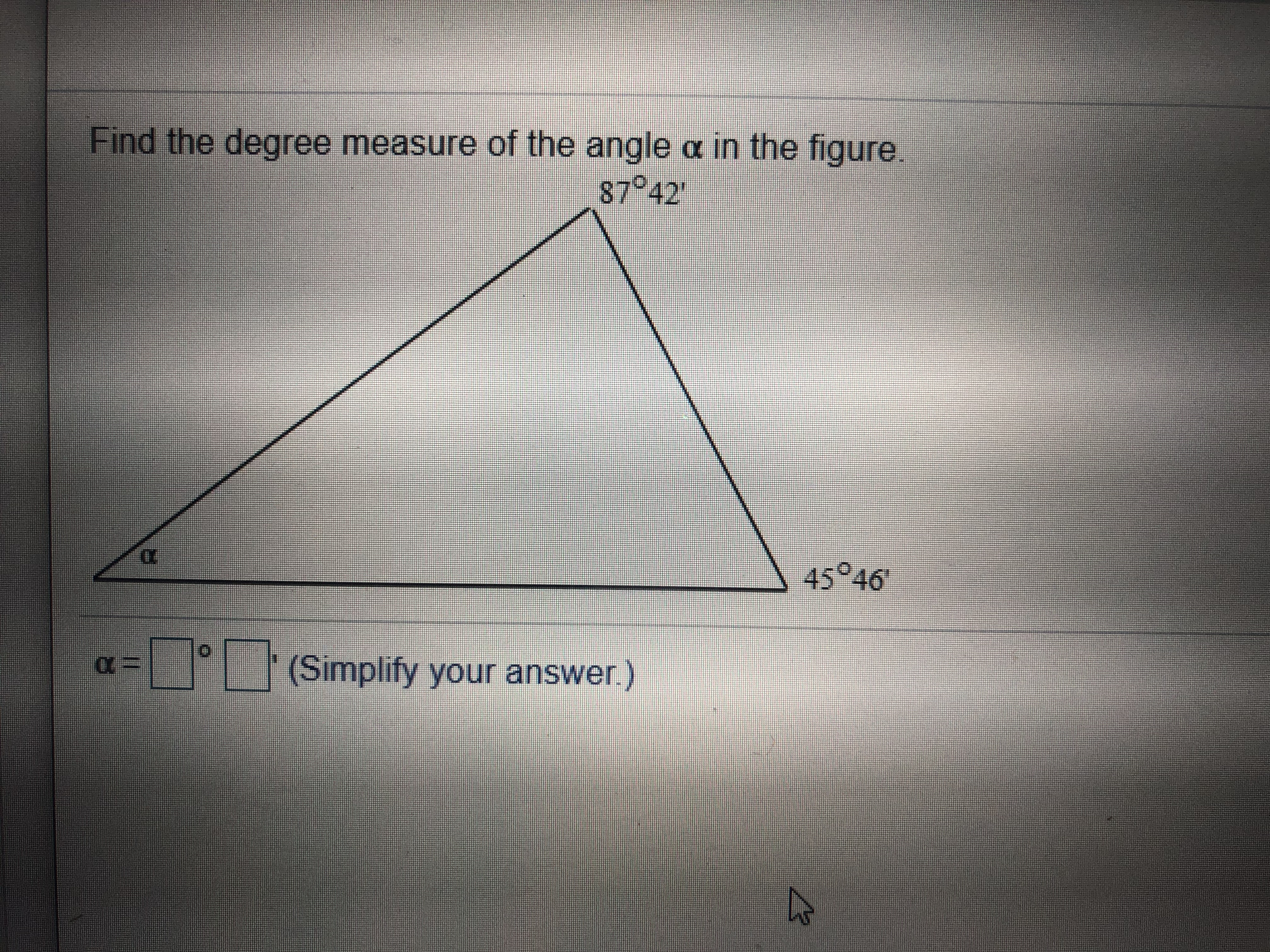Find the degree measure of the angle c in the figure.874245 46(Simplify your answer.)

Question

Can you explain how to figure out this question?help_outlineImage TranscriptioncloseFind the degree measure of the angle c in the figure. 8742 45 46 (Simplify your answer.) fullscreen
Step 1

Consider the given angles.

Step 2

Since, the sum of all three angles of any triangle is 1800.

1 degree = 60 minutes

So, the third angle α will be

180 degree – (sum of two given angle)

Step 3

So, the sum of two g...

Want to see the full answer?

See Solution

Want to see this answer and more?

Our solutions are written by experts, many with advanced degrees, and available 24/7

See Solution
Tagged in

Other